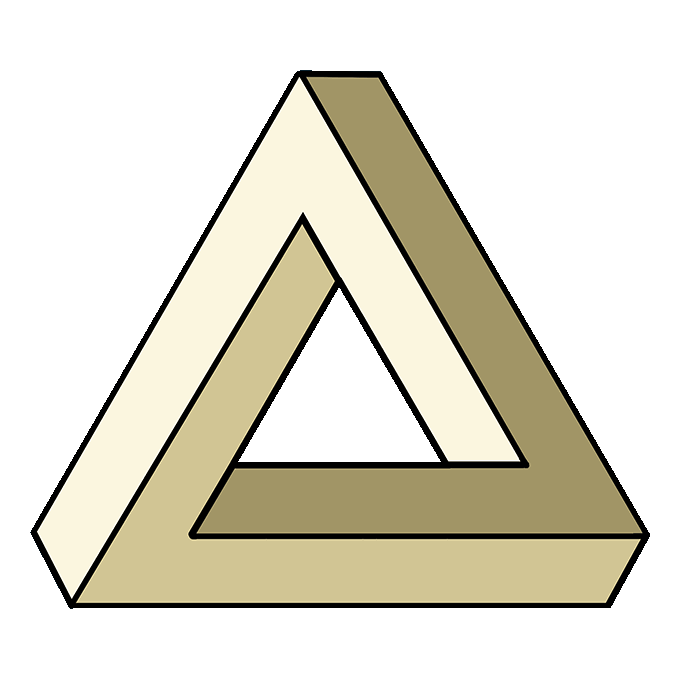# How to Draw the Impossible Triangle

Learn how to draw a great looking Impossible Triangle with easy, step-by-step drawing instructions, and video tutorial.

By following the simple steps, you too can easily draw a perfect Impossible Triangle.​​​​​The impossible triangle, also called the Penrose triangle, infinite triangle, never ending triangle, or impossible tribar, was first drawn by a Swedish artist by the name of Oscar Reutersvärd in 1934.

It was used in the work of psychiatrist Lionel Penrose, mathematician Roger Penrose, and Dutch mathematical artist M.C. Escher, as well as other artists.

The shape has been described as "impossibility in its purest form."

​​​The impossible triangle is a type of optical illusion - an image that, because of the perception of the eyes and brain, differs from reality.

Impossible objects are optical illusions in which a two dimensional figure (something flat, for example, a drawing on paper), is interpreted by the brain to represent a three dimensional object - one that cannot really exist due to its physical proportions.

Look closely at the impossible triangle, and you may see that its sides connect in unusual ways.

​While impossible objects cannot exist in the real world - that's why they are called impossible - artists have put forth efforts to approximate them.

An outdoor sculpture displayed in East Perth, Australia, for example, assumes the appearance of an impossible triangle when viewed from a certain angle. When viewed from other angles, however, it is apparent that the lines of the "triangle" don't actually connect.

​​Psychologists and other scholars have long been interested in optical illusions.

Leonardo da Vinci may have been the first draw at optical illusion, a series of lines that, when you tilted the paper at the correct angle, became an eye.

Researchers say that optical illusions happen because as our brains learn about our surroundings, they begin to make assumptions.

Sometimes these assumptions are wrong, such as when a flat drawing looks three dimensional, and this shift can result in a disconcerted feeling.

​Would you like to draw an impossible triangle? It's not impossible. In fact, it's easy with the help of this simple, step-by-step drawing guide.

All you will need is a pencil, pen, or marker, and a sheet of paper.

You may also wish to use a ruler to help you sketch straight lines, and crayons, colored pencils, or paints to shade your finished drawing.

If you liked this tutorial, see also the following drawing guides: Crystal, Star, and Snowflake.

## ​Step by Step Instructions for Drawing ​the Impossible Triangle

### How to Draw a Great Looking Impossible Triangle for Kids, Beginners, and Adults - Step 11. Begin by drawing a small equilateral triangle, that is, a triangle with sides of equal lengths. This will form the opening in the middle of the impossible triangle.

### Easy Impossible Triangle Drawing - Step 22. Extend a short, straight line diagonally from each of the triangle's points. These lines will form the basis of the impossible triangle's three dimensional appearance.### Easy Impossible Triangle Drawing - Step 33. From the uppermost diagonal line, draw a straight line parallel to the side of the small triangle. Then, from this line, draw another straight line parallel to the bottom of the small triangle.

### Easy Impossible Triangle Drawing - Step 44. Draw a straight, horizontal line from the lowest of the original diagonals. This line should be parallel to and equidistant from the other two horizontal lines.

### Easy Impossible Triangle Drawing - Step 55. Draw a long, straight line upward from the remaining diagonal line, parallel to the side of the small triangle.

### Easy Impossible Triangle Drawing - Step 66. From the uppermost point of the line drawn in the previous step, draw a long, straight line parallel to the opposite side of the triangle.

### Easy Impossible Triangle Drawing - Step 77. From the middle horizontal line, extend a long, straight line upward, parallel to the side of the existing triangle.

### Add More Details to Your Impossible Triangle Picture - Step 88. All that remains is to enclose the figure of the impossible triangle. Begin by closing one of the corners with a short, straight line.

### Complete the Outline of Your Impossible Triangle Drawing - Step 99. Use short, straight lines to enclose the remaining corners of the impossible triangle.

### Color Your Impossible Triangle DrawingReady for more impossible object drawings? One of the easiest impossible shapes to draw is impossible square, this drawing of an impossible star can really confuse your brain, when drawing impossible stairs it's hard to know when the stairs start or end, and you can even draw an impossible heart to your sweetheart.